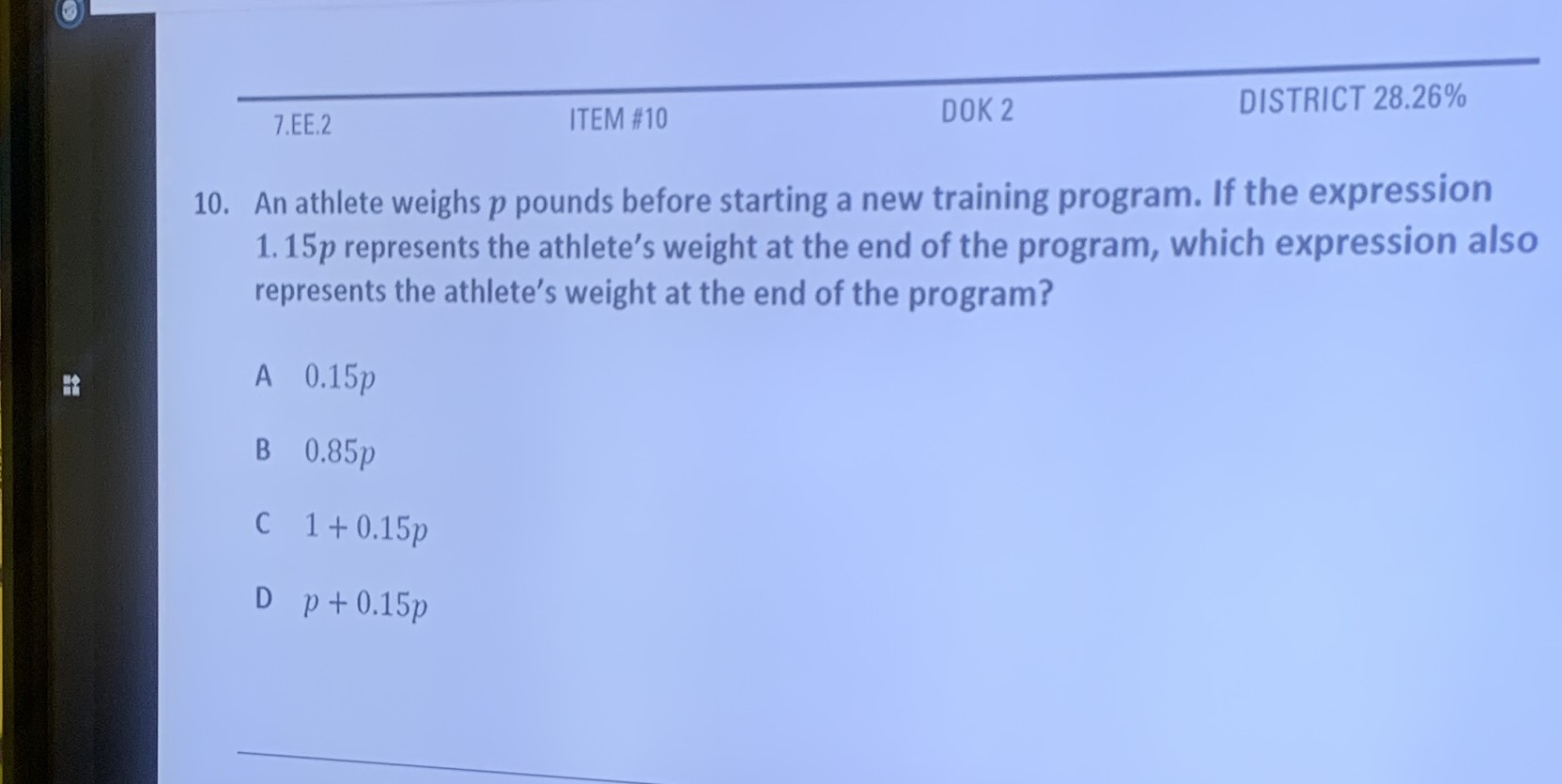### ¿Todavía tienes preguntas de matemáticas?

Pregunte a nuestros tutores expertos
Algebra
Pregunta10. An athlete weighs $$p$$ pounds before starting a new training program. If the expression

$$1.15 p$$ represents the athlete's weight at the end of the program, which expression also represents the athlete's weight at the end of the program?

$$0.15 p$$

$$0.85 p$$

$$1 + 0.15 p$$

$$p + 0.15 p$$